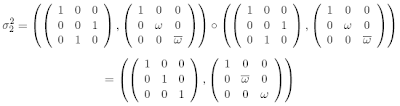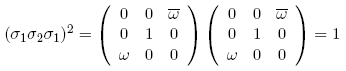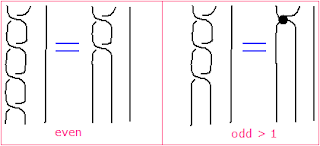Let $\omega$ be the primitive cubed root of unity. Using the ordinary matrix product one finds that the prospective $B_3$ braid generatorsatisfies $\sigma_{2}^{2} = 1$, but as Lieven points out one can consider fancier matrix products, such asand it follows that instead $\sigma_{2}^{3}$ might be a permutation matrix. Anyhow, one easily verifies that the braid relation $\sigma_{1} \sigma_{2} \sigma_{1} = \sigma_{2} \sigma_{1} \sigma_{2}$ holds. Moreover, in this case of cubed roots of unity, using ordinary matrix product one gets the relationwhich reduces the braid group to the modular group. Recall that this process views the group $B_{3}$ as the fundamental group of the complement of the trefoil knot in three dimensional space. Note that the generator $\sigma_{1}$ behaves similarly, reduced by the properties of $\omega$, but never quite to the identity. For powers of $\sigma_{1}$ we have the relationswhere the big dot means the permutation operation, which has no knowledge of the crossing. What a nice way of looking at the modular group! Category theorists have a fancy way of thinking of semidirect products as a piece of two dimensional group structure, but these simple matrices are enough to see what is going on.